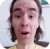FreeKB - Extract fields of data in Perl (split, awk)
Extract fields of data in Perl (split, awk)Split variable into array

Let's say the \$greeting variable has the following text.

``\$greeting = "Hello World How are you today";``

The split operator can be used to create an index of the data. In this example, split is followed by / /, which means that a single white space will be used as the delimter, and the results are stored in array called @strings.

``@strings = split / /, \$greeting;``

When dealing with unpredictible whitespace, use \s+.

``@strings = split /\s+/, \$greeting;``

Dumper can be used to view the results of the split.

``````use Data::Dumper;
print Dumper \@strings;``````

In this example, the is one variable (VAR1) that contains 6 indexes of data.

``````\$VAR1 = [
'Hello',
'World',
'How',
'are',
'you',
'today'
];``````

Now, you can do something will individual pieces of data that were split.

``````print \$strings; # Hello
print \$strings; # World
print \$strings; # How
print \$strings; # are
print \$strings; # you
print \$strings; # today``````

Split variable into new variables

Typically, a variable is split into an array. However, there are situation where it makes more sense to split into new variables. For example, let's say example.txt contains the following text.

``Jeremy Engineer``

This script will store "Jeremy" into a variable called \$name and "Engineer" into a variable called \$occupation.

``````open(FH, '<', "example.txt");
while (<FH>){
my (\$name, \$occupation) = split(/,/);
print "\$name\n";
print "\$occupation\n";
}
close(FH);``````

New lines

If you have a variable that contains new lines, the split operator can be used so that each line of data is it's own chunk of data. Let's say the \$greeting variable contains new lines.

``\$greeting = "Line one\n Line two\n Line three\n Line four\n";``

In this example, /\n/ is used to split the \$greeing variable at new lines, and to then write the results to the @strings array.

``@strings = split /\n/, \$greeting;``

Dumper can again be used to view the results, which shows that each chunk of data is now it's own line.

``````\$VAR1 = [
'Line one',
'Line two',
'Line three',
'Line four'
];``````

Often, a for each loop is then used to iterate each line.

``````foreach (@strings) {
print "\$_\n";
}``````

This will print each lines.

``````Line one
Line two
Line three
Line four``````

If needed, you could then split again inside of the foreach line, to create an index of each piece of data on each line.

``````foreach (@strings) {
@fields = split / /, \$_;
print Dumper \@fields;
}``````

Using Dumper, we can see that each line is now it's own unique variable, and each whitespace separated piece of data is it's own index.

``````\$VAR1 = [
'Line',
'one'
];
\$VAR1 = [
'Line',
'two'
];
\$VAR1 = [
'Line',
'three'
];
\$VAR1 = [
'Line',
'four'
];``````

This will allow us to do something with certain fields of data inside of the foreach loop.

``````foreach (@strings) {
@fields = split / /, \$_;
print "@fields \n";
}``````

In this example, index of each line is printed, which would print the following.

``````one
two
three
four``````

Split a file

Let's say example.txt has the following text.

``Hello World How are you today``

The following Perl script will associate each field of data with a unique variable. In this example, \$field0 would have the value "Hello", \$field1 would have the value "World", and so on.

``````open(FH, '<', example.txt);

while (<FH>) {
\$field0 = ((split ' '));
\$field1 = ((split ' '));
\$field2 = ((split ' '));
\$field3 = ((split ' '));
\$field4 = ((split ' '));
\$field5 = ((split ' '));
}

close(FH);``````

Each variable can then be printed.

``````print "\$field0 \n";
print "\$field1 \n";
print "\$field2 \n";
print "\$field3 \n";
print "\$field4 \n";
print "\$field5 \n";``````

Which would produce the following output.

``````Hello
World
How
are
you
today``````

We will never share your name or email with anyone. Enter your email if you would like to be notified when we respond to your comment.

Please enterin the box below so that we can be sure you are a human.